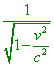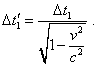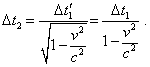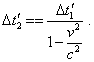SELF 4 S.B. Karavashkin and O.N. KaravashkinaNone the less, the chronologically first postulate which Einstein denied was the principle of full identity of reference frames. To follow the very process, how he denied, consider a standard problem - one of those gedanken experiments which accompanied Einstein’s proof of his views. Let we have two fully identical inertial reference frames K and K' which move with reference to each other with some speed v. On the basis of full equivalence of these reference frames and in accordance with Einstein’s statement of meaningless motion as such, we can state that the observer in K moves in relation to K', and namely so the observer in K' moves in relation to K, and these mutual motions are equivalent. Now suppose, the observer in K decided to check his own clock as follows: in the standard for SR way he passes the time interval to the frame K', where this measure of time is processed and then passed, in the standard way too, back to the observer in K. Implementing this plan, at the first stage the observer in K' does not move and receives the signal from moving observer in K. With it, as the clock moves relative to K' with the speed v, “when observed from this reference frame, not a second will pass between two beats of this clock but(9)
 seconds, i.e. some more time” [12, p. 549]. In other words,(10)
 At the second stage of measurement, the observers interchange their places. Now the observer in K does not move and receives the measure of time from the observer moving in K'. With it, in full accordance with the identity of reference frames, he yields(11)
 Thus, having passed the time interval to the moving observer and receiving from him his own interval, the observer has to see a clear growth of this interval. If the observer in K' conducts similar experiment in parallel, he will yield the same expression (11), but for himself:(12)
 From this, even so-so educated experimenters will unambiguously conclude that in reference frames motion, the time does not transform but the changes are caused by drawback of experimental technique used to check the clocks. This is natural, expected result with the full identity of inertial reference frames. But in accordance with the relativistic conception, the difference in observers exists. “According to special theory of relativity, the coordinate systems K and K' in no way are equivalent. Actually, this theory states the equivalence of only all Galilean (non-accelerated) coordinate systems, i.e. such systems with respect to which enough isolated points move directly and uniformly” [17, p. 618]. But it follows from the said that we may think equivalent only the frames which never accelerated - these surely will be the frames stationary to each other. If between them some speed arose, one or both unavoidably have to accelerate; dependently on, how these frames accelerated before and how they became again inertial, the degree of their non-equivalence will vary. So in the twin paradox, time decelerates at the twin who accelerated. But the cases are quite probable - as for example in the previous problem or in study of celestial bodies - when we simply do not know, which frame accelerated before our study; then, judging by Einstein, we can select such frame by reducing space and time in it. Dividing the reference frames into those which were and were not accelerated before, or were less accelerated, we automatically state that Lorentz transformations are true only in one direction - from a non-accelerated inertial frame to the previously accelerated, not vice versa. Going on, we on the basis of such non-equivalence may try to find a body in the universe which never was accelerated and in relation to it as the absolute frame to calculate the metric transformation of all other bodies. With it, we should not ponder, whether we can reveal such frame experimentally or not, just as Newton was not interesting, in essence, to find experimentally this absolute frame. “It can appear that in reality there does not exist a body at rest to which we might refer the places and motions of others” [6, p. 32]. But even the possibility of such absolute frame contradicts the initial postulate of SR. Thereupon, either reduction of space-time metric is not reality but only a feature of particular technique of measurement of this metric, or the reference frames are non-equivalent and the first postulate appears crushed down by the very author.

Contents / 1 / 2 / 3 / 4 / 5 / 6 / 7 / 8 / 9 / 10 / Paper